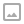## 「NOI2009」二叉查找树-区间dp

·  ✏️ About  1045 words  ·  ☕ 3 mins read · 👀... views

Luogu P1864

## 题解

$$m(l,r,p) = 0; (l \geq r),$$

$$\min_{k = l}^r{(, m(l,k-1,p)+m(k+1,r,p)+ K), ; m(l,k-1,P_k)+m(k+1,r,P_k))+w(i,j) ; ;(l < r)}$$

## 代码

  1 2 3 4 5 6 7 8 9 10 11 12 13 14 15 16 17 18 19 20 21 22 23 24 25 26 27 28 29 30 31 32 33 34 35 36 37 38 39 40 41 42 43 44 45 46 47 48 49 50 51 52 53 54 55 56 57  #include #include #define pp node using namespace std; const int MAXN = 80; int n,K; int sum[MAXN],num[MAXN]; int dp[MAXN][MAXN][MAXN]; struct node{ int fr,sc,tr; bool operator < (const node a)const{ return fr < a.fr; } }; void init(){ scanf("%d %d",&n,&K); pp tmp[MAXN];int t[MAXN]; for(int i = 1;i<=n;i++) scanf("%d",&tmp[i].fr); for(int i = 1;i<=n;i++){ scanf("%d",&tmp[i].sc); t[i] = tmp[i].sc; } for(int i = 1;i<=n;i++) scanf("%d",&tmp[i].tr); sort(tmp+1,tmp+n+1),sort(t+1,t+n+1); for(int i = 1;i<=n;i++){ num[i] = lower_bound(t+1,t+n+1,tmp[i].sc)-t; sum[i] = sum[i-1]+tmp[i].tr; } } void solve(){ int tmp; for(int w = 1;w<=n;w++){ for(int l = 1,r = w;r<=n;l++,r++){ for(int p = 0;p<=n;p++){ dp[l][r][p] = 0x3f3f3f3f; for(int k = l;k<=r;k++){ tmp = min(dp[l][k-1][p] + dp[k+1][r][p] + K, num[k] > p? dp[l][k-1][num[k]] + dp[k+1][r][num[k]]: 0x3f3f3f3f); dp[l][r][p] = min(dp[l][r][p],tmp); } dp[l][r][p] += sum[r] - sum[l-1]; } } } printf("%d\n",dp[n]); } int main(){ init(); solve(); return 0; }WRITTEN BY
cqqqwq
A student in Computer Science.